2d shape

Calculate the content of a shape in which an arbitrary point is not more than 3 cm from the segment AB. The length of the segment AB is 5 cm.

Result

S =  58.274 cm2

Solution:Leave us a comment of example and its solution (i.e. if it is still somewhat unclear...):Be the first to comment!Next similar examples:

1. AnnulusThe radius of the larger circle is 8cm, the radius of smaller is 5cm. Calculate the contents of the annulus.
2. CableCable consists of 8 strands, each strand consists of 12 wires with diameter d = 0.5 mm. Calculate the cross-section of the cable.
3. 22/7 circleCalculate approximately area of a circle with radius 20 cm. When calculating π use 22/7.
4. Annulus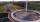Calculate the area of two circles annulus k1 (S, 3 cm) and k2 (S, 5 cm).
5. Circle - easy 2The circle has a radius 6 cm. Calculate:
6. SidewalkThe city park is a circular bed of flowers with a diameter of 8 meters, around it the whole length is 1 meter wide sidewalk . What is the sidewalk area?
7. LawnsBefore a sports hall are two equally large rectangular lawns, each measuring 40 m and 12 m. Maintenance of 10m² lawn cost 45 CZK yearly. On each lawn is circular flowerbed with a diameter 8 meters. How much money is needed each year to take on lawn care?.
8. Tiles path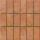A rectangular park measures 24mX18m. A path 2.4m wide is constructed all around it. Find the cost of paving the path with cement tiles of size 60cm X 40cm at the rate of \$5.60 per tile ?
9. Hřiště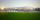Map scale is 1: 5000. The playground is rectangular and on the map has dimensions 10 cm and 5 cm What is area of playground in square meters in reality?
10. Diameter to areaFind the area of a circle whose diameter is 26cm.
11. 6-gon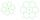Perimeter of regular hexagon is 113. Calculate its circumradius (radius of circumscribed circle).
12. Crocuses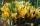The garden grow daffodils, crocuses and roses. 1400 daffodils, crocuses is 462 more and roses is 156 more than crocuses. How many are all the flowers in the garden?
13. Two circlesTwo circles with a radius 4 cm and 3 cm have a center distance 0.5cm. How many common points have these circles?
14. LineCan we construct a line segment, if we know: center
15. Center traverseIt is true that the middle traverse bisects the triangle?Combine like terms 4c+c-7cPlace one pair of brackets in expression 20-16 + 10 - 4 - 2 so that the result will be 0.